We’d like to understand how you use our websites in order to improve them. Register your interest.

# Three-dimensional sediment transport processes on tsunami-induced topography changes in a harbor

## Abstract

A three-dimensional hydrostatic numerical simulation on tsunami-induced topography changes near a harbor is carried out, and sediment transport processes on a significant local deposition near the center of the harbor caused by a tsunami, which was observed in an early experimental study, are investigated. This local deposition has not been well predicted by a vertically averaged hydrodynamic model. The results show that velocities, water levels and topography changes in the harbor predicted in this study agree with the experimental data. The local deposition has relations with a vortex generated in the harbor when the tsunami attacks the harbor. At areas near the vortex center, a secondary flow of the first kind develops, and it plays the role of transporting suspended sediment to the vortex center, located near the center of the harbor, and causes the local deposition there. In order to predict deposition areas with high accuracy, the secondary flow effects should be incorporated in prediction methods of tsunami-induced topography change. Key words: Tsunami, tsunami deposits, sediment transport, hydrodynamic model.

## 1. Introduction

Large tsunamis cause extensive sediment transport in coastal areas. In the past two decades, the tsunami-induced topography changes and the sediment deposition (tsunami deposits), which result from tsunami-induced sediment transport, have become a matter of interest to many geologists and engineers because they are related to tsunami risks (e.g., Dawson and Shi, 2000; Moore et al., 2006; Dawson and Stewart, 2007). A large tsunami transports seabed sediment over large areas and creates deposition of continuous and discontinuous sediment sheets across large areas of the coastal zone (e.g., Hindson etal., 1996; Dawson and Shi, 2000). Thus, tsunami deposits are of geological interest as evidence for the occurrence of past tsunamis and for estimating past tsunami inundation areas. On the other hand, tsunami-induced sediment transport in coastal zones causes local scouring and deposition around coastal facilities. Tomita et al. (2006) reported that extensive erosion was observed around coastal structures and piers in southwest Sri Lanka after the 2004 Indian Ocean tsunami, and as a result their functions and abilities were lost even though damage to the structures themselves was not observed. Thus, tsunami-induced topography changes are of engineering interest that they may adversely affect the ability of coastal facilities.

Tsunamis cause different topography changes than storms in coastal zones because of their different driving forces. Tsunamis have long wavelengths and long wave periods 10 min–1 hour. Thus, tsunami inundation areas are wide and tsunami-induced topography changes occur over larger areas than those caused by wind waves. Furthermore, seabed sediment experiences strong shear stress continuously for a longer time than in the case of wind waves, resulting in tsunami-induced topography changes having different features from storm-induced topography changes.

Numerical models of tsunami-induced topography changes have been developed in a decade (Takahashi et al., 2000; Nishihata et al., 2006; Jaffe and Gelfenbuam, 2007; Huntington et al., 2007; Fujii et al., 2009; Gusman et al., 2010; Huang et al., 2010; Apotsos et al., 2011). These models are classified into two types; inverse models and forward models (Huntington et al., 2007). The inverse models are used to calculate tsunami flow speed from distributions of tsunami deposits. Jaffe and Gelfenbuam (2007) applied an inverse model to a prediction of flow speeds using field data collected at Arop, Papua New Guinea, after the 1998 tsunami. Their model assumes that tsunami-induced sediment transport is under a steady, spatially uniform process and flow speeds are determined by local thickness and grain size of deposits. They showed agreement with estimation by application of Bernoulli’s principle to water levels on buildings and an inundation model.

In the forward models, inundation areas, tsunami flow speeds, and water depths are calculated by hydrodynamic models, and topography changes are calculated by sediment transport models. Goto et al. (2011) calculated the inundation process of the 2004 Indian Ocean tsunami near Kirinda harbor, Sri Lanka, using a two-dimensional vertically averaged hydrodynamic model, and investigated difference observed in bathymetric data one month before and 2 months after the tsunami. Takahashi et al. (2000) and Nishihata et al. (2006) coupled vertically averaged hydrodynamic models and sediment transport models, and carried out numerical simulations of topography changes in Kesen-numa port due to the 1960 Chilean tsunami and those in Kirinda harbor due to the 2004 Indian Ocean tsunami, respectively. In the vertically averaged models, vertical averaged velocities and suspended sediment concentrations are calculated, and vertical profiles of velocity and suspended sediment concentration are given analytically. On the other hand, Apotsos et al. (2011) and Kihara and Matsuyama (2011) applied three dimensional hydrodynamic models with the hydrostatic assumption to estimations of topography changes in Kuala Meurisi, Sumatra, and Kirinda harbor, Sri Lanka, respectively, due to the 2004 Indian Ocean tsunami. Before applying to the sediment transport simulation, Apotsos et al. (2011) carried out a set of benchmark simulations for tsunami run-up, but not for tsunami-induced sediment transport because no standardized benchmarks exists.

Fujii et al. (2009) carried out an experiment using a wide flume in order to clarify characteristic flow patterns and topography changes in harbors due to a tsunami. In their experiment, topography changes near an idealized harbor due to an isolated long wave were investigated. Furthermore, they also carried out numerical simulations on the tsunami-induced topography changes using a vertically averaged model. Their model encountered a difficulty in predicting deposition areas in the harbor. Although a significant local deposition area was observed at the center of the harbor in their experiment, a widespread deposition area was predicted by their numerical model. This inconsistence may have originated from three-dimensional sediment transport in the deposition processes, which cannot be expressed by the vertically averaged model.

In the present study, in order to investigate the deposition processes at the center of the harbor observed in the experiment of Fujii et al. (2009), a three-dimensional hydrostatic numerical simulation is carried out, and we discuss the roles of the three-dimensional sediment transport in the deposition processes. The idealized experiment of Fujii et al. (2009) is an appropriate benchmark for understanding typical tsunami-induced sediment transport processes in harbors or in inner bays, which are important for both geological and engineering aspects because those will be helpful both for searching historical or pre-historical tsunami deposits in inner bays and for safety assessments of coastal structures. This paper is organized as follows: First, Section 2 describes the numerical model used in this study. Then, Section 3 shows the numerical results and a comparison with experimental data. Furthermore, in Section 3, we attempt to clarify the deposition processes on the basis of the results of the numerical simulation.

## 2. Numerical Model

### 2.1 Governing equations for flow

The governing equations for flow are the continuity equations and the momentum equations, with the assumption of the hydrostatic approximation. The equations are transformed into a terrain-following coordinate system (x, y, π) from the Cartesian coordinate system (x, y, z), where x and y denote the horizontal directional coordinates, z denotes the vertical directional coordinate, and π is given as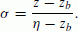((1))

The variables η and z b respectively denote the water level and bed level. From Eq. (1), π = 0 on the bed and π = 1 on the water surface. The governing equations in the terrain-following coordinate system are written as follows:((2))((3))((4))

for i = 1 and 2, where x i = (x, y, π), h is water depth, g is acceleration due to gravity, u i (= (u, v, W)) are horizontal velocities (u,v) and π component of contravariant velocity W, and K i (= (K h , K h , K π )) are horizontal component of the eddy kinematic viscosity K h and π component of eddy kinematic viscosity K π . The π components of the contravariant velocity W and the eddy kinematic viscosity K π have the following relations with the vertical component of the velocity w and the eddy kinematic viscosity K v , respectively: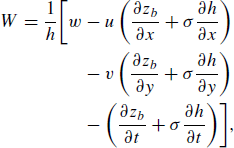((5))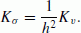((6))

K v is estimated by solving the Mellor-Yamada level-2 closure model (Mellor and Yamada, 1982), and K h is estimated by Smagorinsky model (Smagorinsky, 1963).

### 2.2 Sediment transport equations

The sediment transport is classified into the bed load and the suspended load. The bed load is the sediment movement in the bed load layer, which is a thin layer on the bed, induced by the shear stress, and the suspended load is the sediment movement in the water induced by the mean flow and the turbulence. The bed level is calculated by solving the conservation equation for the mass of the sediment in the bed load layer,((7))

where λ denotes the porosity of the bed sediment and given the value of 0.35 (Garcia, 2008), and w s denotes the settling velocity of the sediment in the water and is given by Soulsby’s formula (Soulsby, 1997). Variables C b , E b , and q bi (i = x and y) are the suspended sediment concentrationat the reference level a, which is the top of the bed load layer, the entrainment rate of the suspended sediment into the water, and the volumetric bed load transport rate in the i direction, respectively. E b and q b are estimated using the van Rijn’s formulae (van Rijn, 1984a, b),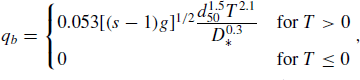((8))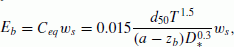((9))

where C eq is the equilibrium concentration at the reference level a, s (= 2.65) is the specific density of sediment, and d50 is the median diameter of sediment. Variables T and D* are the dimensionless excess shear stress and dimensionless grain size, defined as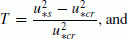((10))((11))

where v is the kinetic viscosity of water, u*s is the efficient friction velocity on the bed, and u*cr is the critical friction velocity. The total stress on the bedis composed of the friction dragand the form drag τbf, where ρ w is the density of water. The friction drag contributes to the sediment motion on the bed and is estimated by the formula of Celik and Rodi (1991).((12))

To estimate the critical friction velocity u*cr, an algebraic expression of based on the Shields diagram proposed by Iwagaki (1956) is used. The formulation of the reference level a has been studied in decades because the level directly related to magnitude of the entrainment rate, as shown in (9) (e.g., van Rijn, 1984b; Garcia and Parker, 1991). In the early studies, the level a is given as a function of water depth, diameter of bed material, or Niku-radse equivalent roughness height, but we think there is no formula which is applicable in any situations. In this study, the reference level a is assumed to be proportional to the Nikuradse equivalent roughness height k s (= d50), i.e., a = c α × k s , and the coefficient c α is chosen by fitting the magnitude of predicted suspended sediment concentration in the test case described in Subsection 2.5 with experimental results. As a result, c α = 1.0 is used in this study.

The volumetric suspended sediment C is calculated by solving the conservation equation for the suspended sediment in the water,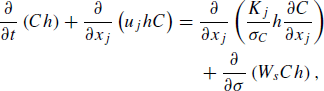((13))

where W s is the contravariant settling velocity, and σ C is the turbulent Schmidt number and is set to be 1.0 following Wu et al. (2000).

### 2.3 Boundary conditions

By assuming the velocity near the bed to be distributed logarithmically in the vertical direction, the boundary conditions for the horizontal velocities and the friction velocity are given as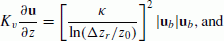((14))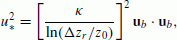((15))

where Δ r is the distance between the near-bed layer level and the bed level, u b is the horizontal velocity at the near-bed layer, κ (= 0.41) is the Karman coefficient, and z0 is the roughness height, given as k s /30. From the definition of W, W on the bed becomes zero. Neglecting the wind shear on the water surface, the boundary condition for the horizontal velocity at the water level is given as((16))

W at the water level also becomes zero.

The entrainment rate of the suspended sediment in the water E b is equals to the turbulent flux of the suspended sediment in the vertical direction at the reference level a:((17))

On the water surface, the total suspended sediment flux in the vertical direction is zero on the water surface;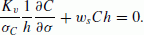((18))

### 2.4 Numerical method

In the numerical simulation, the discretized governing equations (2)(4), (7), and (13) are solved using the finite-difference method on staggered grids. The first-order up wind differential scheme is used for the advection terms and the second-order central differential scheme is used for the other terms. For the time integration of these equations, the free-surface correction method proposed by Chen (2003) is used. In this method, a semi-implicit scheme is used for the time integration of the vertical diffusion terms and terms including the water level, and an explicit scheme is used for the other terms to allow a long time interval.

### 2.5 Validation of the numerical model

In order to validate our numerical model, a well-documented test case is simulated (van Rijn, 1986; Wu et al., 2000; Liang et al., 2005). The case was experimentally studied by van Rijn (1981), and the experimental data was available. In the experiment, a straight flume with 30 m length, 0.5 m width, and 0.7 m height was used, and the generation of concentration profiles in a clear water flow was investigated. Figure 1(a) shows a schematic diagram of the experiment. The median diameter of the bed material d50 was 0.23 mm, and a rigid flat surface was set upstream of a movable bed. The water depth h was 0.25 m, and the mean flow velocity was 0.67 m/s. During the test period, small deformations of the bed form were generated at the surface of the movable bed (height 0.015 m, length 0.1 m), and the test period was as short as possible to maintain the uniformity of the flow.

In the numerical test, the computational domain is a two-dimensional with a flat bed, as shown in Fig. 1(b). A vertically uniform flow with a velocity of 0.67 m/s is given as the inflow boundary condition at x = −15 m, and a water depth of 0.25 m is given as the outflow boundary condition at x = 15 m. A sediment bed is set in the region of 0 m ≤ x ≤ 15 m and the median diameter of the bed material is 0.23 mm. Although suspended sediment is generated over the sediment bed, the bed form is assumed not to be deformed since the bed form deformation in the experiment was small and negligible. In the region upstream of the sediment bed region, a rigid flat surface from which suspended sediment is not generated is present. In Fig. 2, vertical profiles of predicted suspended sediment concentration at various points with x / h = 4, 10, 20, and 40 are compared with the experimental data. Agreement can be observed among them, thus validating our numerical model for the diffusion of suspended sediment.

## 3. Tsunami-Induced Topography Change in a Harbor

In this section, a three-dimensional hydrostatic numerical simulation of the tsunami-induced topography changes studied experimentally by Fujii et al. (2009) is carried out, and the deposition process at the center of the harbor is discussed.

### 3.1 Experiment of Fujii et al. (2009)

In the experiment of Fujii et al. (2009), a harbor with 6.0 m length × 6.0 m width was set on the sediment bed in a wide flume (58 m length, 20 m width, 1.6 m depth), as shown in Fig. 3. The harbor had two breakwaters; the breakwater located on the offshore side was called the exterior breakwater and the other breakwater was called the interior breakwater. The median diameter of the bed material d50 was 0.08 mm. The still water depth at the harbor was 0.08 m. An isolated long wave with 60 s period and 0.06 cm wave height was generated at the opposite side of the harbor by a wave maker and traveled toward the harbor. By controlling the velocity at the wave maker, the influence of the reflection at the wave maker was reduced and the topography changes caused by only one long wave thus could be investigated in the experiment. There was not wave breaking or overtopping in their experiment.

### 3.2 Numerical conditions

The numerical domains used in this study are shown in Fig. 4. In the numerical simulation, to simultaneously calculate tsunami propagation in the long flume and the tsunami-induced sediment transport in the harbor in detail, a nested grid system with four domains of different resolutions is used. The velocity, the water depth, the suspended sediment concentration and the topography at lateral boundaries are exchanged among each domain. The harbor is set in domain 3. In domains 2, 3 and 4, the bed is movable and the median diameter of the bed material is 0.08 mm, although the bed is rigid in domain 1. The horizontal grid resolutions (Δx, Δy) are uniform for each domain, and they are 0.225 m for domain 1, 0.075 m for domain 2, 0.025 m for domain 3, and 0.0083 m for domain 4. The vertical grid resolution (Δσ) is varied and the resolution becomes finer as approaching the bed. The minimum vertical grid size (Δz) in the harbor for the still water is 0.0015 m and the maximum vertical grid size is 0.0067 m. The grid numbers in the horizontal directions (N x × N y ) are 129 × 87 for domain 1, 90 × 33 for domain 2, 150 × 81 for domain 3, and 78 × 93 for domain 4. The number of vertical layers is 18. The long wave is generated by giving a time series of water levels, which were measured near the wave maker in the experiment, as a boundary condition at x = 29.7 m. The free slip condition is applied to the side boundaries and the cell face between the fluid cell and the solid cell.

### 3.3 Numerical results and comparisons with experimental data

Figure 5 shows snapshots of the vertically averaged velocity vectors at t = 26 s, 36 s, and 51 s in the harbor, and Fig. 6 shows those of the water levels. At t = 26 s, the long wave approaches the harbor, and a fast flow with a velocity of 1.2 m/s is observed near the head of the interior breakwater, which is driven by the water level difference between the inside and outside of the harbor. A horizontal wake vortex is generated behind the interior breakwater. The wake vortex is advected to the center of the harbor (Fig. 5(b)), and the vortex flow appears to circulate in the harbor. At the center of the vortex, the local lowest water level is observed (Figs. 6(a) and (b)). At t = 51 s, the water level in the harbor is higher than that outside of the harbor, and owing to this water level difference, the wave-induced flow returns offshore. In Figs. 7 and 8, the velocities and water levels at the three points in the harbor specified in Fig. 3 predicted in the present study are compared with the experimental data. The velocities are measured at a height of 4 cm above the bed. From these figures, good agreement is observed among the measured and predicted values. The flow behaviors predicted in this study is also similar to those predicted by the vertically averaged model.

Topography changes predicted in this study are shown in Fig. 9. The topography changes measured by the laboratory experiments and predicted by the vertically averaged model by Fujii et al. (2009) are also shown. The experimental data shows erosion near the heads of breakwaters and local deposition near the center of the harbor. Both the topography changes predicted in this study and those predicted by the vertically averaged model show erosion near the heads of breakwaters and are in agreement with experimental data.

The erosion depths near the exterior and interior breakwaters predicted in this study are 1.7 cm and 3.7 cm, and those predicted by the vertically averaged model are 9.4 cm and 5.5 cm, though those observed in the experiment are 5.5 cm and 6.3 cm, respectively. On the other hand, this study predicts local deposition near the center of the harbor, in agreement with the experimental data, whereas the vertically averaged model predicts widespread deposition areas in the harbor as explained in Section 1. This indicates that the local deposition near the center of the harbor may be caused by three-dimensional sediment transport. Note that the deposition height observed in the experiment is 1.3 cm, but those predicted in this study is 0.57 cm and predicted by the vertically averaged model is 0.63 cm, and the both numerical simulations underestimate the deposition height. In the following subsection, the sediment transport processes on the local deposition at the center of the harbor are discussed through the analysis of our numerical results.

### 3.4 Sediment transport processes on the local deposition at the center of the harbor

Figures 10 and 11 respectively show vertically averaged suspended sediment and budget of suspended sediment near the bed at t = 26 s and 36 s. The budget of suspended sediment near the bed is the difference between the mass of suspended sediment entrained into the water from the bed and that deposited from the water onto the bed. The Shields numberof the vortex flow in the harbor is 0.3−1.5 at t = 26 s and 36 s and the particle Reynolds number Re p (= (sgd50)1/2d50/v) is 2.88; thus, Parker’s diagram (Garcia, 2000) shows that suspended load is dominant rather than bedload in the sediment transport induced by the vortex flow at t = 26 s and 36 s. Therefore, the budget of suspended sediment near the bed can be used as an index denoting deposition/erosion, and a positive budget value at a point denotes that erosion is occurring there, and a negative budget value at a point denotes that deposition is occurring there.

At t = 26 s, when the long wave approaches the harbor, a large amount of suspended sediment is generated near the head of the interior breakwater and erosion occurs there (Fig. 11(a)). The suspended sediment is advected in the direction of the flow (Figs. 10(a) and (b)). A high concentration of suspended sediment is observed near the vortex center but the local lowest concentration is observed just at the vortex center at t = 26 s and 36 s. Near the vortex center, which is close to the center of the harbor at t = 36 s, local deposition is observed (Fig. 11(b)). As shown in Fig. 3, the vertically averaged flow in the harbor appears to circulate in the harbor, and thus, the suspended sediment does not tend to be transported toward the vortex center if it is transported along the streamlines of the vertically averaged flow. In the following, we discuss how the suspended sediment is transported toward the vortex center.

Note that at areas with curved streamlines such as those of the vortex flow, an Ekman layer develops near the bed and a secondary flow of the first kind is generated owing to the balance of the centrifugal force and pressure gradient (e.g., Melling and Whitelaw, 1976). This phenomenon of the secondary flow is well known as the “teapot effects” playing the role of transporting sediment around the center part of the circulation (Kimura et al., 2010). Here, we investigate presence of the secondary flow in our numerical results. The velocities u is decomposed into stream-wise components u s , which parallels with the vertically averaged flow direction, and cross-components u c , which are perpendicular to the vertically averaged flow direction, i.e., u = u s +u c . The spatial distribution of the cross-component of the velocity near the bed (2 mm above the bed) at t = 36 s is shown in Fig. 12. From Fig. 12, flow directed toward the vortex center, i.e., a secondary flow, can be observed in the harbor. Due to the presence of the secondary flow, the direction of the near-bed flow is clockwise declined toward the vortex center in the harbor.

Here, we compare strength of the secondary flow observed in this study with those estimated by the method proposed by Kalkwijk and de Vriend (1980) in which strength of secondary flow is guessed by using vertically averaged velocity. The strength of secondary flow A n observed in this study is estimated by assuming that vertical profile of velocity of the secondary flow component is expressed as ǀu c ǀ = A n ǀ f (z)ǀ, where f (z) is a profile function. Using a profile function f (z) = 2(z/h − 1/2) (Odgaard, 1989), A n is calculated by using the cross-components of velocity near the bed shown in Fig. 12.

The strength of secondary flow A n estimated by the method of Kalkwijk and de Vriend (1980) is simply calculated by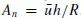, whereis a vertically averaged velocity and R is a curvature radius of streamlines. The strength of secondary flow at t = 36 s estimated by the above-mentioned two methods are compared in Fig. 13. To understand the figures easily, values at only areas where the vertically averaged velocity is larger than 0.1 m/s are shown in the figure. Difference of spatial distributions of A n is observed between the two method, although order of magnitude of A n is the same among them. This would be because the vortex which causes the secondary flow is unsteady, so that flow profiles of the secondary flow is not determined only by instantaneous states of flow.

In order to evaluate impact of the secondary flow on suspended sediment transport toward the vortex center, the mass of suspended sediment advected by the secondary flow toward the vortex center is estimated in the following way. Vertically integrated mass of suspended sediment advected by flow is estimated by. Advection flux of suspended sediment Cu is decomposed as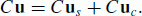((19))

The second term of the right hand side of (19) denotes advection flux of suspended sediment owing to the secondary flow. Near the vortex center, the direction of the secondary flow near the bed is 90° to the right of the vertically averaged flow direction (cf. Figs. 5(b) and 12). Thus, the vertically integrated mass of suspended sediment advected owing to the secondary flow toward the vortex center is expressed as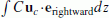, where erightward is a unit vector with 90° to the right of the vertically averaged flow direction. The spatial distribution of ratio of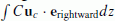toat t = 36 s is shown in Fig. 13. To understand the figure easily, values at only areas where the vertically averaged velocity is larger than 0.1 m/s are shown in the figure. As increasing the distance from the vortex center, the ratio increases, and it partially becomes larger than 0.1. As increasing the distance further, the ratio decreases. The figure shows that, near the vortex center, the secondary flow has the role of transporting the 5–10% of suspended sediment toward the vortex center. As approaching the vortex center, the velocity becomes lower, thus the suspended sediment tends to be deposited near the vortex center. It is also interesting that the roles of a secondary flow in suspended sediment transport are remarkable near the head of the interior breakwater.

## 4. Conclusions

A three-dimensional hydrostatic numerical simulation on tsunami-induced topography changes near a harbor was carried out, and the deposition processes at the center of the harbor were investigated. The velocity, water level and topography changes in the harbor predicted by our numerical model agree with experimental data. The deposition at the center of the harbor could be predicted by our numerical model, although it could not be well predicted by the vertically averaged numerical model. This is because a secondary flow of the first kind, which was generated near the vortex and developed in the harbor, plays the role of transporting suspended sediment to the vortex center, which is located near the center of the harbor.

Such a vortex has actually been witnessed in harbors after a tsunami (e.g., Okal et al., 2006). Numerical simulations of the topography changes near Kirinda harbor in Sri Lanka induced by the 2004 Indian Ocean tsunami using our numerical model show that some vortices were generated near Kirinda harbor when the tsunami inundated around the harbor, and some deposition areas were observed near the centers of the vortices, in agreement with field survey data (Kihara and Matsuyama, 2011). Thus, to predict deposition areas with high accuracy, the secondary flow effects should be incorporated in numerical models.

The results obtained in this study will be helpful for searching historical or pre-historical tsunami deposits in inner bays. There is a high potential that tsunami deposits are preserved in inner bays where ocean wave influence is weak and thus topography changes due to sediment transports by the ocean waves were little after tsunamis (Fujiwara et al., 2000; Goto et al., 2011). Our results show that depositions induced by tsunamis would be occurred at areas where long-duration sustaining vortices are generated and strong return flows are not occurred. Such vortices would be generated behind peninsulas, which play similar roles to breakwaters in the harbor shown in this study.

## References

1. Apotsos, A., M. Buckley, G. Gelfenbaum, B. Jaffe, and D. Vat-vani, Nearshore tsunami inundation model validation: Toward sediment transport applications, Pure Appl. Geophys., doi:10. 1007/s00024.011.0291.5, 2011.

2. Celik, I. and W. Rodi, Suspended sediment transport capacity for open channel flow, J. Hydraul. Eng., 114, 191–204, 1991.

3. Chen, X., A free-surface correction method for simulating shallow water flows, J. Comput. Phys., 189, 557–578, 2003.

4. Dawson, A. G. and S. Shi, Tsunami deposits, Pure Appl. Geophys., 157, 875–897, 2000.

5. Dawson, A. G. and I. Stewart, Tsunami deposits in the geological record, Sediment. Geol., 200, 166–183, 2007.

6. Fujii, N., M. Ikeno, T. Sakakiyama, M. Matsuyama, M. Takao, and T. Mukouhara, Hydraulic experiment on flow and topography change in harbor due to tsunami and its numerical simulation, Ann. J. Coast. Eng., 56, 291–295, 2009 (in Japanese).

7. Fujiwara, O., F. Masuda, T. Sakai, T. Irizuki, and K. Fuse, Tsunami deposits in Holocene bay mud in southern Kanto region, Pacific coast of central Japan, Sediment. Geol., 135, 219–230, 2000.

8. Garcia, M. H., Discussion of “The Legend of A. F. Shields”, J. Hydraul. Eng., 126, 718–720, 2000.

9. Garcia, M. H., Sedimentation Engineering Processes, Measurements, Modeling and Practice, 1050 pp., ASCE, Reston, Va, 2008.

10. Garcia, M. H. and G. Parker, Entrainment of bed sediment into suspension, J. Hydraul. Eng., 117, 414–435, 1991.

11. Goto, K., J. Takahashi, T. Oie, and F. Imamura, Remarkable bathymetric change in the nearshore zone by the 2004 Indian Ocean tsunami: Kirinda Harbor, Sri Lanka, Geomorphology, 127, 107–116, 2011.

12. Gusman, A. R., Y. Tanioka, and T. Takahashi, Modeling of the sediment transport by the 2004 Sumatra-Andaman tsunami in Lhoknga, Banda Aceh, Indonesia, Proceeding of 3rd International Tsunami Field Symposium, 33–34, 2010.

13. Hindson, R. A., C. Andrade, and A. G. Dawson, Sedimentary processes associated with the tsunami generated by the 1755 Lisbon earthquake on the Algarve coast, Portugal, Phys. Chem. Earth, 21, 57–63, 1996.

14. Huang, Z., L. Li, and Q. Qui, A numerical study of tsunami-induced erosion along the coast of Painan, Indonesia, Proceeding of 3rd International Tsunami Field Symposium, 183–184, 2010.

15. Huntington, K., J. Bourgeois, G. Gelfenbaum, P. Lynett, B. Jaffe, H. Yeh, and R. Weiss, Sandy signs of a tsunami’s onshore depth and speed, Eos Trans., 82, 577–578, 2007.

16. Iwagaki, Y., Fundamental study on critical tractive force, Trans. JSCE, 41, 1–21, 1956.

17. Jaffe, B. E. and G. Gelfenbuam, A simple model for calculating tsunami flow speed from tsunami deposits, Sediment. Geol., doi:10.1016/j.sedgeo.2007.01.013, 2007.

18. Kalkwijk, J. P. T. and H. J. de Vriend, Computation of the flow in shallow river bends, J. Hydraul. Res., 18, 327–342, 1980.

19. Kihara, N. and M. Matsuyama, Numerical simulations of sediment transport induced by the 2004 Indian Ocean tsunami near Kirinda port in Sri Lanka, Proceedings of 32th International Conference on Coastal Engineering, currents.12, 2011.

20. Kimura, I., S. Onda, T. Hosoda, and Y. Shimizu, Computations of suspended sediment transport in a shallow side-cavity using depth-averaged 2D models with effects of secondary currents, J. Hydro-Environ. Res., 4, 153–161, 2010.

21. Liang, D., L. Cheng, and F. Li, Numerical modeling of flow and scour below a pipeline in currents Part II. Scour simulation, Coast. Eng., 52, 43–62, 2005.

22. Melling, A. and J. H. Whitelaw, Turbulent flow in a rectangular duct, J. Fluid Mech., 78, 289–315, 1976.

23. Mellor, G. L. and T. Yamada, Development of a turbulence closure model for geophysical fluid problems, Rev. Geophys. Space Phys., 20, 851– 875, 1982.

24. Moore, A., Y. Nishimura, G. Gelfenbaum, T. Kamataki, and R. Triyono, Sedimentary deposits of the 26 December 2004 tsunami on the northwest coast of Aceh, Indonesia, Earth Planets Space, 58, 253–258, 2006.

25. Nishihata, T., Y. Tajima, Y. Moriya, and T. Sekimoto, Topography change due to the Dec 2004 Indian Ocean Tsunami—Field and numerical study at Kirinda port, Sri Lanka, Proceedings of 30th International Conference on Coastal Engineering, 1456–1468, 2006.

26. Odgaard, A. J., River-meander model: I. Development, J. Hydraul. Eng., 115, 1433–1450, 1989.

27. Okal, E. A., H. M. Fritz, P. E. Raad, C. Synolakis, Y. Al-Shijbi, and M. Al-Saifi, Oman field survey after the December 2004 Indian Ocean Tsunami, Earthq. Spectra, 22, S203–S218, 2006.

28. Smagorinsky, J., General circulation experiments with the primitive equations, Mon. Weath. Rev., 91, 99–164, 1963.

29. Soulsby, R., Dynamics of Marine Sands, Thomas Telford, Ltd., 270 p., 1997.

30. Takahashi, T., N. Shuto, F. Imamura, and D. Asai, Modeling sediment transport due to tsunamis with exchange rate between bed load layer and suspended load layer, Proceedings of the 27th International Conference on Coastal Engineering, 1508–1519, 2000.

31. Tomita, T., F. Imamura, T. Arikawa, T. Yasuda, and Y. Kawata, Damage caused by the 2004 Indian Ocean Tsunami on the southwestern coast of Sri Lanka, Coast. Eng. J., 48, 99–116, 2006.

32. Van Rijn, L. C., Experience with straight flumes for movable bed experiments, IAHR Workshop on particle motion, 1981.

33. Van Rijn, L. C., Sediment transport, Part I: Bed load transport, J. Hydraul. Eng., 110, 1431–1456, 1984a.

34. Van Rijn, L. C., Sediment transport, Part II: Suspended load transport, J. Hydraul. Eng., 110, 1613–1641, 1984b.

35. Van Rijn, L. C., Mathematical modeling of suspended sediment in nonuni-form flows, J. Hydraul. Eng., 112, 433–455, 1986.

36. Wu, W., W. Rodi, and T. Wenka, 3D numerical modeling of flow and sediment transport in open channels, J. Hydraul. Eng., 126, 4–15, 2000.

## Acknowledgments

We would like to thank Dr. Goto and Dr. Takahashi who gave us invaluable comments and suggestions, which led to significant improvements of our paper.

## Author information

Authors

### Corresponding author

Correspondence to Naoto Kihara.

## Rights and permissions

Reprints and Permissions

Kihara, N., Fujii, N. & Matsuyama, M. Three-dimensional sediment transport processes on tsunami-induced topography changes in a harbor. Earth Planet Sp 64, 1 (2012). https://doi.org/10.5047/eps.2011.05.036

• Revised:

• Accepted:

• Published:

### Key words

• Tsunami
• tsunami deposits
• sediment transport
• hydrodynamic model